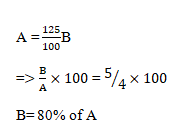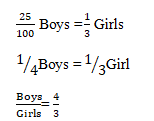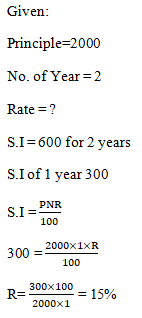# RRB ALP 2018 Practice Test Papers | Arithmetic Questions (Day-46)

Dear Aspirants, Here we have given the Important RRB ALP & Technicians Exam 2018 Practice Test Papers. Candidates those who are preparing for RRB ALP 2018 can practice these Arithmetic Questions to get more confidence to Crack RRB 2018 Examination.

[WpProQuiz 2593]

Click “Start Quiz” to attend these Questions and view Explanation

1. Find the factors of (x2 – x – 42)?
1. (X+6)(X−6)
2. (X−6) (X−7)
3. (X+6) (X−7)
4. (X+6) (X+7)
1. Sagul got married to Alma 8 years ago. Today his age is 11/4 times of his age at the time of his marriage. Find his age at the time of marriage.
1. 10
2. 15
3. 22
4. 25
1. Find the mean value of 2,3,2,9,2,4, 6,7,7?
1. 5.89
2. 4.67
3. 4.69
4. 5.67
1. A’s income is 25% more than B’s income. B’s income is what percent of A’s income?
1. 60%
2. 80%
3. 70%
4. 75%
1. In a Music Class, 25% of Boys are same in number as 1/3rd of the Girls. What is the ratio of boys to Girls in that Music Class?
1. 5:4
2. 4: 5
3. 4:3
4. 3:4
1. The simple interest on Rs. 2000 for 2 years at Rs.600. What is the Rate of Interest?
1. 10%
2. 20
3. 15%
4. 30%

7.Akshaya sold a table for Rs. 330 and thus lost 12%. At what price he should have sold it to gain 12%?

1. 420
2. 422
3. 424
4. 428
1. Sankar can do piece of work in 18 days, Savita is 50% more efficient than Sankar. How much more times does Sankar take than savita to do the same piece of work?
1. 3
2. 6
3. 4
4. 2
1. A train running at 180 kmph overtakes a car running at 72 kmph in 50 seconds. What is the length of the train in meters?
1. 1200m
2. 1500m
3. 1600m
4. 1800m
1. The area of a square is 144 sq cm, find length of its diagonal?
1. 12
2. 12√2
3. 13√2
4. 13

X2 − X – 42

= X2−7X + 6X – 42

= X (X−7)+ 6(X−7)

= (X+6) (X−7)

Let the present age of Sagul = X years

8years ago, his age was = (X – 8) years

According to given condition,

× (X – 8) = X

11 × (X – 88) = 4X

11X – 88 = 7X

4X = 88

X = 22

Age of Sagul at his marriage is 22 years.

Sum of the given numbers = 2 + 3+2 +9+ 2 + 4 + 6 + 7 +7 = 42

Mean of the given values = 42/9 = 4.67Time taken by Sankar to the Savita = 150 : 100

Let x be the number of days taken by Sankar to do a piece of work

=>150 : 100 = 3:2

⇒ x = 2 × 18/3 = 12

Sankar takes 18 – x = 18 – 12 = 6 times than Savitato do the same piece of work.

Length of the train = Relative speed between the train and the car × time taken

Relative speed of the train = (180 – 72) = 108 × 5/18 = 30 (∵ they are travelling in same direction)

∴ Length of the train = 30 × 50 = 1500 m

Length of a diagonal = √2 × side

Given, area of a square is 100 sq cm

⇒ side2 = 144

⇒side = 12 cm

Length of a diagonal = √2×12

= 12√2

RRB ALP 2018 – “All in One” Study Materials and Practice Sets